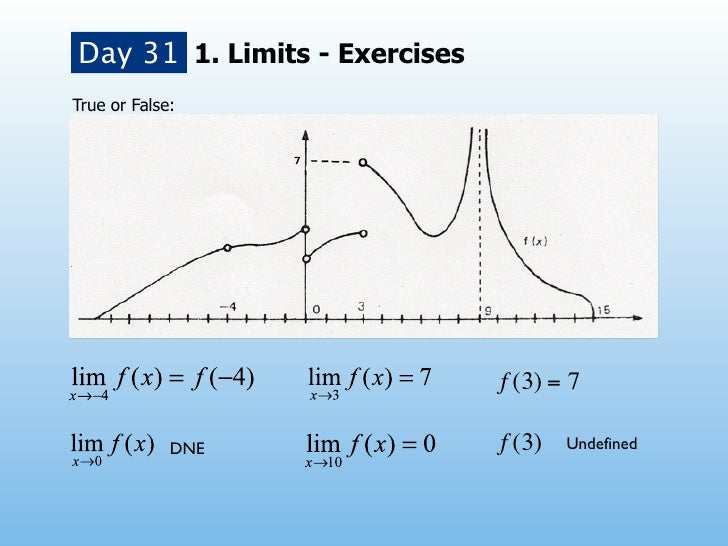Successfully reported this slideshow.Upcoming SlideShare
×

# PM5006 Week 7

704 views

Published on

Aquí están las transparencias de la semana 7 de mi curso de Cálculo diferencial. Algunas transparencias las tomé de Dan Meyer y Dan Greene.

Published in: Education
• Full Name
Comment goes here.

Are you sure you want to Yes No• Be the first to like this

### PM5006 Week 7

1. 1. Day 31 1. Limits - ExercisesTrue or False: ! f (3) = 7 DNE ! f (3) Undefined
2. 2. Day 31 1. Limits - ExercisesTrue or False: ! f (3) = 7 False DNE ! f (3) Undefined
3. 3. Day 31 1. Limits - ExercisesTrue or False: ! f (3) = 7 False False DNE ! f (3) Undefined
4. 4. Day 31 1. Limits - ExercisesTrue or False: ! f (3) = 7 False False False DNE ! f (3) Undefined
5. 5. Day 31 1. Limits - ExercisesTrue or False: ! f (3) = 7 False False False DNE ! f (3) Undefined True
6. 6. Day 31 1. Limits - ExercisesTrue or False: ! f (3) = 7 False False False DNE ! f (3) Undefined True False
7. 7. Day 31 1. Limits - ExercisesTrue or False: ! f (3) = 7 False False False DNE ! f (3) Undefined True False True
8. 8. 1. Limits - ExercisesTrue or False: ! f (0) = 5 DNE f (3) Undefined
9. 9. 1. Limits - ExercisesTrue or False: ! f (0) = 5 False DNE f (3) Undefined
10. 10. 1. Limits - ExercisesTrue or False: ! f (0) = 5 False False DNE f (3) Undefined
11. 11. 1. Limits - ExercisesTrue or False: ! f (0) = 5 False False True DNE f (3) Undefined
12. 12. 1. Limits - ExercisesTrue or False: ! f (0) = 5 False False True DNE f (3) Undefined True
13. 13. 1. Limits - ExercisesTrue or False: ! f (0) = 5 False False True DNE f (3) Undefined True True
14. 14. 1. Limits - ExercisesTrue or False: ! f (0) = 5 False False True DNE f (3) Undefined True True False
15. 15. 2. NotesFind the following limit 2 lim 2x − 3x − 4 x→−1
16. 16. 2. NotesFind the following limit 2 lim 2x − 3x − 4 x→−1
17. 17. 3. ExercisesFind the following limits1. lim x 1 x→− 32. lim π x→−1 3x + 73. lim x→4 x + 1 x + 154. lim x→4 3 5− x5. lim x→−6 2
18. 18. 3. ExercisesFind the following limits1. lim x 1 x→− 3 -1/32. lim π x→−1 3x + 73. lim x→4 x + 1 x + 154. lim x→4 3 5− x5. lim x→−6 2
19. 19. 3. ExercisesFind the following limits1. lim x 1 x→− 3 -1/32. lim π x→−1 3x + 73. lim 19/5 x→4 x + 1 x + 154. lim x→4 3 5− x5. lim x→−6 2
20. 20. 3. ExercisesFind the following limits1. lim x 1 x→− 3 -1/32. lim π x→−1 π 3x + 73. lim 19/5 x→4 x + 1 x + 154. lim x→4 3 5− x5. lim x→−6 2
21. 21. 3. ExercisesFind the following limits1. lim x 1 x→− 3 -1/32. lim π x→−1 π 3x + 73. lim 19/5 x→4 x + 1 x + 15 194. lim x→4 3 3 5− x5. lim x→−6 2
22. 22. 3. ExercisesFind the following limits1. lim x 1 x→− 3 -1/32. lim π x→−1 π 3x + 73. lim 19/5 x→4 x + 1 x + 15 194. lim x→4 3 3 5− x 115. lim x→−6 2 2
23. 23. Day 321. LimitsFind the following limit 2 x −1 lim x→1 x − 1
24. 24. Day 321. LimitsFind the following limit 2 x −1 lim x→1 x − 1
25. 25. 1. LimitsFind the following limits 2 x − 5x − 61. lim x→6 x−6 3 x −12. lim x→1 x − 1
26. 26. 1. LimitsFind the following limit x +1 lim x→−1 3 − x + 10
27. 27. 2. Limits - ExercisesFind the following limits: 3h1. lim h→0 h x−32. lim 2 x→3 x − 9 2 x + 7x3. lim x→0 x x +14. lim x→−1 3 − x + 10 2 25. lim ( x + h) −x h→0 h
28. 28. Day 331. LimitsFind the following limit 2 x −1 lim x→1 x − 1
29. 29. Day 331. LimitsFind the following limit 2 x −1 lim x→1 x − 1